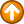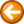Int - return the integer portion of a decimal number\$return = int(decimal_number)

Where:

 \$return receives the integer portion of the decimal number.
 decimal_number is a decimal number.

decimal_number may be a variable.

The int command returns the integer portion of a decimal number.

Examples

\$result = int(1.5)

\$result = int(\$number)